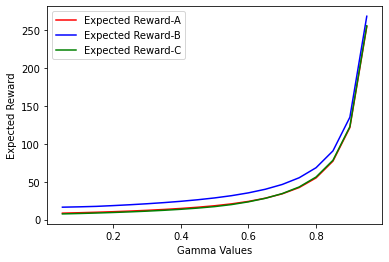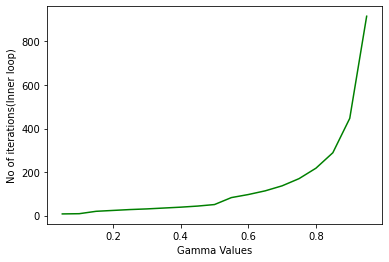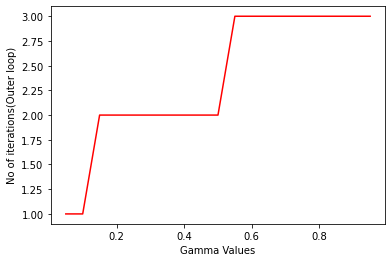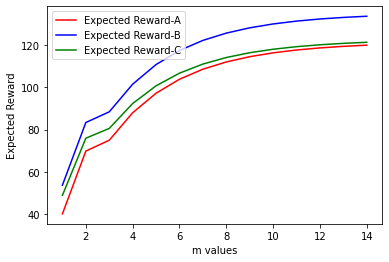# Solution for submission 132273

A detailed solution for submission 132273 submitted for challenge IIT-M RL-ASSIGNMENT-2-TAXI

# What is the notebook about?¶

## Problem - Taxi Environment Algorithms¶

This problem deals with a taxi environment and stochastic actions. The tasks you have to do are:

• Implement Policy Iteration
• Implement Modified Policy Iteration
• Implement Value Iteration
• Implement Gauss Seidel Value Iteration
• Visualize the results
• Explain the results

## How to use this notebook? 📝¶

• This is a shared template and any edits you make here will not be saved.You should make a copy in your own drive. Click the "File" menu (top-left), then "Save a Copy in Drive". You will be working in your copy however you like.

• Update the config parameters. You can define the common variables here

Variable Description
AICROWD_DATASET_PATH Path to the file containing test data. This should be an absolute path.
AICROWD_RESULTS_DIR Path to write the output to.
AICROWD_ASSETS_DIR In case your notebook needs additional files (like model weights, etc.,), you can add them to a directory and specify the path to the directory here (please specify relative path). The contents of this directory will be sent to AIcrowd for evaluation.
AICROWD_API_KEY In order to submit your code to AIcrowd, you need to provide your account's API key. This key is available at https://www.aicrowd.com/participants/me

# Setup AIcrowd Utilities 🛠¶

We use this to bundle the files for submission and create a submission on AIcrowd. Do not edit this block.

In :
!pip install aicrowd-cli > /dev/null


# AIcrowd Runtime Configuration 🧷¶

Get login API key from https://www.aicrowd.com/participants/me

In :
import os
import numpy as np
AICROWD_DATASET_PATH = os.getenv("DATASET_PATH", os.getcwd()+"/13d77bb0-b325-4e95-a03b-833eb6694acd_a2_taxi_inputs.zip")
AICROWD_RESULTS_DIR = os.getenv("OUTPUTS_DIR", "results")

In :


API Key valid
Saved API Key successfully!
13d77bb0-b325-4e95-a03b-833eb6694acd_a2_taxi_inputs.zip: 100% 31.2k/31.2k [00:00<00:00, 255kB/s]

In :
!unzip $AICROWD_DATASET_PATH  Archive: /content/13d77bb0-b325-4e95-a03b-833eb6694acd_a2_taxi_inputs.zip replace inputs/inputs_base.npy? [y]es, [n]o, [A]ll, [N]one, [r]ename: A inflating: inputs/inputs_base.npy inflating: inputs/inputs_1.npy inflating: inputs/inputs_0.npy inflating: inputs/inputs_2.npy inflating: targets/targets_2.npy inflating: targets/targets_0.npy inflating: targets/targets_1.npy inflating: targets/targets_base.npy  In : DATASET_DIR = 'inputs/'  ## Taxi Environment¶ Read the environment to understand the functions, but do not edit anything In : import numpy as np class TaxiEnv_HW2: def __init__(self, states, actions, probabilities, rewards, initial_policy): self.possible_states = states self._possible_actions = {st: ac for st, ac in zip(states, actions)} self._ride_probabilities = {st: pr for st, pr in zip(states, probabilities)} self._ride_rewards = {st: rw for st, rw in zip(states, rewards)} self.initial_policy = initial_policy self._verify() def _check_state(self, state): assert state in self.possible_states, "State %s is not a valid state" % state def _verify(self): """ Verify that data conditions are met: Number of actions matches shape of next state and actions Every probability distribution adds up to 1 """ ns = len(self.possible_states) for state in self.possible_states: ac = self._possible_actions[state] na = len(ac) rp = self._ride_probabilities[state] assert np.all(rp.shape == (na, ns)), "Probabilities shape mismatch" rr = self._ride_rewards[state] assert np.all(rr.shape == (na, ns)), "Rewards shape mismatch" assert np.allclose(rp.sum(axis=1), 1), "Probabilities don't add up to 1" def possible_actions(self, state): """ Return all possible actions from a given state """ self._check_state(state) return self._possible_actions[state] def ride_probabilities(self, state, action): """ Returns all possible ride probabilities from a state for a given action For every action a list with the returned with values in the same order as self.possible_states """ actions = self.possible_actions(state) ac_idx = actions.index(action) return self._ride_probabilities[state][ac_idx] def ride_rewards(self, state, action): actions = self.possible_actions(state) ac_idx = actions.index(action) return self._ride_rewards[state][ac_idx]  ## Example of Environment usage¶ In : def check_taxienv(): # These are the values as used in the pdf, but they may be changed during submission, so do not hardcode anything states = ['A', 'B', 'C'] actions = [['1','2','3'], ['1','2'], ['1','2','3']] probs = [np.array([[1/2, 1/4, 1/4], [1/16, 3/4, 3/16], [1/4, 1/8, 5/8]]), np.array([[1/2, 0, 1/2], [1/16, 7/8, 1/16]]), np.array([[1/4, 1/4, 1/2], [1/8, 3/4, 1/8], [3/4, 1/16, 3/16]]),] rewards = [np.array([[10, 4, 8], [ 8, 2, 4], [ 4, 6, 4]]), np.array([[14, 0, 18], [ 8, 16, 8]]), np.array([[10, 2, 8], [6, 4, 2], [4, 0, 8]]),] initial_policy = {'A': '1', 'B': '1', 'C': '1'} env = TaxiEnv_HW2(states, actions, probs, rewards, initial_policy) print("All possible states", env.possible_states) print("All possible actions from state B", env.possible_actions('B')) print("Ride probabilities from state A with action 2", env.ride_probabilities('A', '2')) print("Ride rewards from state C with action 3", env.ride_rewards('C', '3')) base_kwargs = {"states": states, "actions": actions, "probabilities": probs, "rewards": rewards, "initial_policy": initial_policy} return base_kwargs base_kwargs = check_taxienv() env = TaxiEnv_HW2(**base_kwargs)  All possible states ['A', 'B', 'C'] All possible actions from state B ['1', '2'] Ride probabilities from state A with action 2 [0.0625 0.75 0.1875] Ride rewards from state C with action 3 [4 0 8]  ## Task 1 - Policy Iteration¶ Run policy iteration on the environment and generate the policy and expected reward In : # 1.1 Policy Iteration def policy_iteration(taxienv, gamma): # A list of all the states states = taxienv.possible_states # Initial values values = {s: 0 for s in states} # This is a dictionary of states to policies -> e.g {'A': '1', 'B': '2', 'C': '1'} policy = taxienv.initial_policy.copy() ## Begin code here # Hints - # Do not hardcode anything # Only the final result is required for the results # Put any extra data in "extra_info" dictonary for any plots etc # Use the helper functions taxienv.ride_rewards, taxienv.ride_probabilities, taxienv.possible_actions # For terminating condition use the condition exactly mentioned in the pdf J = {s: 0 for s in states} pi = taxienv.initial_policy.copy() outerLoopCount = 0 innerLoopCount = 0 while(True): outerLoopCount = outerLoopCount+1 while(True): D = 0 for s in states: j = J[s] jVal = 0 for sp in states: sp_idx = states.index(sp) acn = pi[s] prob = taxienv.ride_probabilities(s, acn)[sp_idx] rew = taxienv.ride_rewards(s, acn)[sp_idx] jVal = jVal + prob*(rew + gamma*J[sp]) # print(s, sp, sp_idx, acn, prob, rew, J[s]) J[s] = jVal D = max(D, abs(j-J[s])) # print("36, ", D) innerLoopCount = innerLoopCount + 1 if(D < (1e-8)): break done = 1 # print("39, ", pi) for s in states: b = pi[s] psblAcns = taxienv.possible_actions(s) bestAcn = psblAcns bestVal = -10000 for acn in psblAcns: val = 0 for sp in states: sp_idx = states.index(sp) prob = taxienv.ride_probabilities(s, acn)[sp_idx] rew = taxienv.ride_rewards(s, acn)[sp_idx] val = val + prob*(rew + gamma*J[sp]) if(val > bestVal): bestVal = val bestAcn = acn pi[s] = bestAcn if(b != pi[s]): # print("61, ", b, pi[s], s) done = 0 if(done == 1): break values = J policy = pi # Put your extra information needed for plots etc in this dictionary extra_info = {} extra_info['outerLoopCount'] = outerLoopCount extra_info['innerLoopCount'] = innerLoopCount ## Do not edit below this line # Final results return {"Expected Reward": values, "Policy": policy}, extra_info  # Task 2 - Policy Iteration for multiple values of gamma¶ Ideally this code should run as is In : # 1.2 Policy Iteration with different values of gamma def run_policy_iteration(env): gamma_values = np.arange(5, 100, 5)/100 results, extra_info = {}, {} for gamma in gamma_values: results[gamma], extra_info[gamma] = policy_iteration(env, gamma) return results, extra_info results, extra_info = run_policy_iteration(env)  # Task 3 - Modifed Policy Iteration¶ Implement modified policy iteration (where Value iteration is done for fixed m number of steps) In : # 1.3 Modified Policy Iteration def modified_policy_iteration(taxienv, gamma, m): # A list of all the states states = taxienv.possible_states # Initial values values = {s: 0 for s in states} # This is a dictionary of states to policies -> e.g {'A': '1', 'B': '2', 'C': '1'} policy = taxienv.initial_policy.copy() ## Begin code here # Hints - # Do not hardcode anything # Only the final result is required for the results # Put any extra data in "extra_info" dictonary for any plots etc # Use the helper functions taxienv.ride_rewards, taxienv.ride_probabilities, taxienv.possible_actions # For terminating condition use the condition exactly mentioned in the pdf J = {s: 0 for s in states} pi = taxienv.initial_policy.copy() while(True): for k in range(m): for s in states: jVal = 0 for sp in states: sp_idx = states.index(sp) acn = pi[s] prob = taxienv.ride_probabilities(s, acn)[sp_idx] rew = taxienv.ride_rewards(s, acn)[sp_idx] jVal = jVal + prob*(rew + gamma*J[sp]) # print(s, sp, sp_idx, acn, prob, rew, J[s]) J[s] = jVal done = 1 # print("39, ", pi) for s in states: b = pi[s] psblAcns = taxienv.possible_actions(s) bestAcn = psblAcns bestVal = -10000 for acn in psblAcns: val = 0 for sp in states: sp_idx = states.index(sp) prob = taxienv.ride_probabilities(s, acn)[sp_idx] rew = taxienv.ride_rewards(s, acn)[sp_idx] val = val + prob*(rew + gamma*J[sp]) if(val > bestVal): bestVal = val bestAcn = acn pi[s] = bestAcn if(b != pi[s]): # print("61, ", b, pi[s], s) done = 0 if(done == 1): break values = J policy = pi # Put your extra information needed for plots etc in this dictionary extra_info = {} ## Do not edit below this line # Final results return {"Expected Reward": values, "Policy": policy}, extra_info  # Task 4 Modified policy iteration for multiple values of m¶ Ideally this code should run as is In : def run_modified_policy_iteration(env): m_values = np.arange(1, 15) gamma = 0.9 results, extra_info = {}, {} for m in m_values: results[m], extra_info[m] = modified_policy_iteration(env, gamma, m) return results, extra_info results, extra_info = run_modified_policy_iteration(env) # print(results)  # Task 5 Value Iteration¶ Implement value iteration and find the policy and expected rewards In : # 1.4 Value Iteration def value_iteration(taxienv, gamma): # A list of all the states states = taxienv.possible_states # Initial values values = {s: 0 for s in states} # This is a dictionary of states to policies -> e.g {'A': '1', 'B': '2', 'C': '1'} policy = taxienv.initial_policy.copy() ## Begin code here # Hints - # Do not hardcode anything # Only the final result is required for the results # Put any extra data in "extra_info" dictonary for any plots etc # Use the helper functions taxienv.ride_rewards, taxienv.ride_probabilities, taxienv.possible_actions # For terminating condition use the condition exactly mentioned in the pdf J = {s: 0 for s in states} H = {s: 0 for s in states} pi = taxienv.initial_policy.copy() while(True): D = 0 for s in states: psblAcns = taxienv.possible_actions(s) bestAcn = psblAcns bestVal = -10000000 for acn in psblAcns: val = 0 for sp in states: sp_idx = states.index(sp) prob = taxienv.ride_probabilities(s, acn)[sp_idx] rew = taxienv.ride_rewards(s, acn)[sp_idx] val = val + prob*(rew + gamma*J[sp]) if(val >= bestVal): bestVal = val bestAcn = acn H[s] = bestVal pi[s] = bestAcn D = max(D, abs(J[s]-H[s])) for s in states: J[s] = H[s] if(D < (1e-8)): break values = J policy = pi # Put your extra information needed for plots etc in this dictionary extra_info = {} ## Do not edit below this line # Final results return {"Expected Reward": values, "Policy": policy}, extra_info  # Task 6 Value Iteration with multiple values of gamma¶ Ideally this code should run as is In : def run_value_iteration(env): gamma_values = np.arange(5, 100, 5)/100 results = {} results, extra_info = {}, {} for gamma in gamma_values: results[gamma], extra_info[gamma] = value_iteration(env, gamma) return results, extra_info results, extra_info = run_value_iteration(env)  # Task 7 Gauss Seidel Value Iteration¶ Implement Gauss Seidel Value Iteration In : # 1.4 Gauss Seidel Value Iteration def gauss_seidel_value_iteration(taxienv, gamma): # A list of all the states # For Gauss Seidel Value Iteration - iterate through the values in the same order states = taxienv.possible_states # Initial values values = {s: 0 for s in states} # This is a dictionary of states to policies -> e.g {'A': '1', 'B': '2', 'C': '1'} policy = taxienv.initial_policy.copy() # Hints - # Do not hardcode anything # For Gauss Seidel Value Iteration - iterate through the values in the same order as taxienv.possible_states # Only the final result is required for the results # Put any extra data in "extra_info" dictonary for any plots etc # Use the helper functions taxienv.ride_rewards, taxienv.ride_probabilities, taxienv.possible_actions # For terminating condition use the condition exactly mentioned in the pdf ## Begin code here J = {s: 0 for s in states} pi = taxienv.initial_policy.copy() while(True): D = 0 for s in states: j = J[s] psblAcns = taxienv.possible_actions(s) bestAcn = psblAcns bestVal = -10000000 for acn in psblAcns: val = 0 for sp in states: sp_idx = states.index(sp) prob = taxienv.ride_probabilities(s, acn)[sp_idx] rew = taxienv.ride_rewards(s, acn)[sp_idx] val = val + prob*(rew + gamma*J[sp]) if(val > bestVal): bestVal = val bestAcn = acn J[s] = bestVal pi[s] = bestAcn D = max(D, abs(j - J[s])) if(D < (1e-8)): break values = J policy = pi # Put your extra information needed for plots etc in this dictionary extra_info = {} ## Do not edit below this line # Final results return {"Expected Reward": values, "Policy": policy}, extra_info  # Task 8 Gauss Seidel Value Iteration with multiple values of gamma¶ Ideally this code should run as is In : def run_gauss_seidel_value_iteration(env): gamma_values = np.arange(5, 100, 5)/100 results = {} results, extra_info = {}, {} for gamma in gamma_values: results[gamma], extra_info[gamma] = gauss_seidel_value_iteration(env, gamma) return results, extra_info results, extra_info = run_gauss_seidel_value_iteration(env) print(results)  {0.05: {'Expected Reward': {'A': 8.511527294546923, 'B': 16.400259909029575, 'C': 7.4988690667106095}, 'Policy': {'A': '1', 'B': '1', 'C': '1'}}, 0.1: {'Expected Reward': {'A': 9.076506149392834, 'B': 16.85636856362452, 'C': 8.050865123980312}, 'Policy': {'A': '1', 'B': '1', 'C': '1'}}, 0.15: {'Expected Reward': {'A': 9.70812149293256, 'B': 17.464503042356245, 'C': 8.66916045411395}, 'Policy': {'A': '1', 'B': '2', 'C': '1'}}, 0.2: {'Expected Reward': {'A': 10.43703007363936, 'B': 18.48214285510763, 'C': 9.384398495833036}, 'Policy': {'A': '1', 'B': '2', 'C': '1'}}, 0.25: {'Expected Reward': {'A': 11.274074072135488, 'B': 19.62962962714145, 'C': 10.207407406785403}, 'Policy': {'A': '1', 'B': '2', 'C': '1'}}, 0.3: {'Expected Reward': {'A': 12.243837240938772, 'B': 20.934065930435885, 'C': 11.162756161665108}, 'Policy': {'A': '1', 'B': '2', 'C': '1'}}, 0.35: {'Expected Reward': {'A': 13.3787144345583, 'B': 22.43076922870161, 'C': 12.28282402456309}, 'Policy': {'A': '1', 'B': '2', 'C': '1'}}, 0.4: {'Expected Reward': {'A': 14.722222217898851, 'B': 24.166666661495807, 'C': 13.611111109029693}, 'Policy': {'A': '1', 'B': '2', 'C': '1'}}, 0.45: {'Expected Reward': {'A': 16.33413126434644, 'B': 26.20553359041331, 'C': 15.207370703537933}, 'Policy': {'A': '1', 'B': '2', 'C': '1'}}, 0.5: {'Expected Reward': {'A': 18.298701293837997, 'B': 28.63636363077955, 'C': 17.155844153026397}, 'Policy': {'A': '1', 'B': '2', 'C': '1'}}, 0.55: {'Expected Reward': {'A': 20.78998863222275, 'B': 31.60739669290458, 'C': 19.830725212339292}, 'Policy': {'A': '1', 'B': '2', 'C': '2'}}, 0.6: {'Expected Reward': {'A': 24.02568643692515, 'B': 35.32772363671855, 'C': 23.458813101474426}, 'Policy': {'A': '1', 'B': '2', 'C': '2'}}, 0.65: {'Expected Reward': {'A': 28.276692061545695, 'B': 40.09628057546244, 'C': 28.129978786496867}, 'Policy': {'A': '1', 'B': '2', 'C': '2'}}, 0.7: {'Expected Reward': {'A': 34.06193076743594, 'B': 46.43541615465501, 'C': 34.36604101132381}, 'Policy': {'A': '1', 'B': '2', 'C': '2'}}, 0.75: {'Expected Reward': {'A': 42.31741137784243, 'B': 55.28505390328474, 'C': 43.10631739208525}, 'Policy': {'A': '1', 'B': '2', 'C': '2'}}, 0.8: {'Expected Reward': {'A': 55.079365051578144, 'B': 68.5582010300939, 'C': 56.26984124730355}, 'Policy': {'A': '2', 'B': '2', 'C': '2'}}, 0.85: {'Expected Reward': {'A': 77.24651209951202, 'B': 90.81170061982581, 'C': 78.43345572578708}, 'Policy': {'A': '2', 'B': '2', 'C': '2'}}, 0.9: {'Expected Reward': {'A': 121.65347104963263, 'B': 135.30627544959796, 'C': 122.83690300915262}, 'Policy': {'A': '2', 'B': '2', 'C': '2'}}, 0.95: {'Expected Reward': {'A': 255.0229082673451, 'B': 268.7646183445272, 'C': 256.20284927787594}, 'Policy': {'A': '2', 'B': '2', 'C': '2'}}}  # Generate Results ✅¶ In : # Do not edit this cell def get_results(kwargs): taxienv = TaxiEnv_HW2(**kwargs) policy_iteration_results = run_policy_iteration(taxienv) modified_policy_iteration_results = run_modified_policy_iteration(taxienv) value_iteration_results = run_value_iteration(taxienv) gs_vi_results = run_gauss_seidel_value_iteration(taxienv) final_results = {} final_results["policy_iteration"] = policy_iteration_results final_results["modifed_policy_iteration"] = modified_policy_iteration_results final_results["value_iteration"] = value_iteration_results final_results["gauss_seidel_iteration"] = gs_vi_results return final_results  In : # Do not edit this cell, generate results with it as is if not os.path.exists(AICROWD_RESULTS_DIR): os.mkdir(AICROWD_RESULTS_DIR) for params_file in os.listdir(DATASET_DIR): kwargs = np.load(os.path.join(DATASET_DIR, params_file), allow_pickle=True).item() results = get_results(kwargs) idx = params_file.split('_')[-1][:-4] np.save(os.path.join(AICROWD_RESULTS_DIR, 'results_' + idx), results)  # Check your local score¶ This score is not your final score, and it doesn't use the marks weightages. This is only for your reference of how arrays are matched and with what tolerance. In : # Check your score on the given test cases (There are more private test cases not provided) target_folder = 'targets' result_folder = AICROWD_RESULTS_DIR def check_algo_match(results, targets): param_matches = [] for k in results: param_results = results[k] param_targets = targets[k] policy_match = param_results['Policy'] == param_targets['Policy'] rv = [v for k, v in param_results['Expected Reward'].items()] tv = [v for k, v in param_targets['Expected Reward'].items()] rewards_match = np.allclose(rv, tv, rtol=3) equal = rewards_match and policy_match param_matches.append(equal) return np.mean(param_matches) def check_score(target_folder, result_folder): match = [] for out_file in os.listdir(result_folder): res_file = os.path.join(result_folder, out_file) results = np.load(res_file, allow_pickle=True).item() idx = out_file.split('_')[-1][:-4] # Extract the file number target_file = os.path.join(target_folder, f"targets_{idx}.npy") targets = np.load(target_file, allow_pickle=True).item() algo_match = [] for k in targets: algo_results = results[k] algo_targets = targets[k] algo_match.append(check_algo_match(algo_results, algo_targets)) match.append(np.mean(algo_match)) return np.mean(match) if os.path.exists(target_folder): print("Shared data Score (normalized to 1):", check_score(target_folder, result_folder))  Shared data Score (normalized to 1): 0.9910714285714286  ## Visualize results of Policy Iteration with multiple values of gamma¶ Add code to visualize the results In : ## Visualize policy iteration with multiple values of gamma # 1.2 Policy Iteration with different values of gamma def run_policy_iteration(env): gamma_values = np.arange(5, 100, 5)/100 results, extra_info = {}, {} for gamma in gamma_values: results[gamma], extra_info[gamma] = policy_iteration(env, gamma) return results, extra_info results, extra_info = run_policy_iteration(env) # print(results) gamma_arr = list(np.arange(5, 100, 5)/100) outerLoopCount_arr = [] innerLoopCount_arr = [] expRewA = [] expRewB = [] expRewC = [] for g in gamma_arr: outerLoopCount_arr.append(extra_info[g]['outerLoopCount']) innerLoopCount_arr.append(extra_info[g]['innerLoopCount']) expRewA.append(results[g]['Expected Reward']['A']) expRewB.append(results[g]['Expected Reward']['B']) expRewC.append(results[g]['Expected Reward']['C']) # print(outerLoopCount_arr) # print(innerLoopCount_arr) # print(gamma_arr) import matplotlib.pyplot as plt plt.plot(gamma_arr, expRewA, 'r', label = "Expected Reward-A") plt.plot(gamma_arr, expRewB, 'b', label = "Expected Reward-B") plt.plot(gamma_arr, expRewC, 'g', label = "Expected Reward-C") plt.legend(loc='upper left') plt.xlabel('Gamma Values') plt.ylabel('Expected Reward') plt.show() plt.plot(gamma_arr, innerLoopCount_arr, 'g') plt.xlabel('Gamma Values') plt.ylabel('No of iterations(Inner loop)') plt.show() plt.plot(gamma_arr, outerLoopCount_arr, 'r') plt.xlabel('Gamma Values') plt.ylabel('No of iterations(Outer loop)') plt.show()# Subjective questions¶ ## 1.a How are values of$\gamma$affecting results of policy iteration¶ Modify this cell/add code for your answer. ### Answer:¶ 1. The rate of convergence decreases quiet quickly as gamma increases. 2. On the bright side, higher gamma results in larger expected rewards. ## 1.b For modified policy itetaration, do you find any improvement if you choose m=10.¶ ### Answer¶ As we can see from the below graph, the rate of increse in expected reward decreases as we increase 'm' value. From the code, we can see that the runtime increases linearly with 'm' value. Therefore, although a very high value of 'm' results in better expected rewards, the runtime will also be very high for a little increase in expected reward and is not worth it. In : def run_modified_policy_iteration(env): m_values = np.arange(1, 15) gamma = 0.9 results, extra_info = {}, {} for m in m_values: results[m], extra_info[m] = modified_policy_iteration(env, gamma, m) return results, extra_info results, extra_info = run_modified_policy_iteration(env) # print(results) import matplotlib.pyplot as plt m_values_arr = list(np.arange(1, 15)) expRewA = [] expRewB = [] expRewC = [] for m in m_values_arr: expRewA.append(results[m]['Expected Reward']['A']) expRewB.append(results[m]['Expected Reward']['B']) expRewC.append(results[m]['Expected Reward']['C']) plt.plot(m_values_arr, expRewA, 'r', label = "Expected Reward-A") plt.plot(m_values_arr, expRewB, 'b', label = "Expected Reward-B") plt.plot(m_values_arr, expRewC, 'g', label = "Expected Reward-C") plt.legend(loc='upper left') plt.xlabel('m values') plt.ylabel('Expected Reward') plt.show()## 1.c Compare and contrast the behavior of Value Iteration and Gauss Seidel Value Iteraton¶ ### Answer¶ If we observe both the algorithms, we can observe that they are very similar, with the difference being, in normal VI, New values (H(s)'s) are computed for all the states and J values are updated to H at the end of computing H(s) for all the states. Whereas in Gauss-Seidel VI, the newly computed values (J(s)'s) will be immediately used in the computation of values of other states in the same iteration itself. This leads to faster convergance and lesser runtime for Gauss-Seidel VI # Submit to AIcrowd 🚀¶ In [ ]: !DATASET_PATH=$AICROWD_DATASET_PATH aicrowd notebook submit -c iit-m-rl-assignment-2-taxi -a assets

No jupyter lab module found. Using jupyter notebook.
Using notebook: /content/CS17B021_T1_Assignment_2_Taxi_Release.ipynb for submission...
Removing existing files from submission directory...
No jupyter lab module found. Using jupyter notebook.
Scrubbing API keys from the notebook...
Collecting notebook...
No jupyter lab module found. Using jupyter notebook.
Validating the submission...
Executing install.ipynb...
[NbConvertApp] Converting notebook /content/submission/install.ipynb to notebook
[NbConvertApp] Executing notebook with kernel: python3
[NbConvertApp] Writing 1055 bytes to /content/submission/install.nbconvert.ipynb
Executing predict.ipynb...
[NbConvertApp] Converting notebook /content/submission/predict.ipynb to notebook
[NbConvertApp] Executing notebook with kernel: python3
[NbConvertApp] ERROR | unhandled iopub msg: colab_request
[NbConvertApp] ERROR | unhandled iopub msg: colab_request
[NbConvertApp] ERROR | unhandled iopub msg: colab_request
[NbConvertApp] ERROR | unhandled iopub msg: colab_request
[NbConvertApp] ERROR | unhandled iopub msg: colab_request
[NbConvertApp] ERROR | unhandled iopub msg: colab_request
[NbConvertApp] Writing 38336 bytes to /content/submission/predict.nbconvert.ipynb
submission.zip ━━━━━━━━━━━━━━━━━━━━━━ 100.0% • 28.3/26.7 KB • 2.0 MB/s • 0:00:00
╭─────────────────────────╮
│ Successfully submitted! │
╰─────────────────────────╯
┌──────────────────┬───────────────────────────────────────────────────────────────────────────────────────────────────────────────────────────┐
│  This submission │ https://www.aicrowd.com/challenges/iit-m-assignment-2/problems/iit-m-rl-assignment-2-taxi/submissions/132159              │
│                  │                                                                                                                           │
│  All submissions │ https://www.aicrowd.com/challenges/iit-m-assignment-2/problems/iit-m-rl-assignment-2-taxi/submissions?my_submissions=true │
│                  │                                                                                                                           │